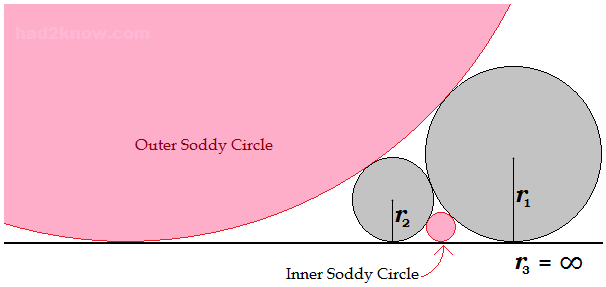# Descartes' Theorem for Two Tangent Circles and a Line

### Calculating the Radius of a Circle Tangent to Two Given Circles and a Line

Inner Soddy Circle Radius =
Outer Soddy Circle Radius =

If you have two circles that are tangent to each other and mutually tangent to a line, you can use Descartes' Theorem to calculate the radius of another circle that is mutually to all three objects. This is done by considering the line to be a circle of infinite radius, transforming the original problem into the traditional Soddy Circle Problem.

Just as in the regular Soddy Circle Problem, there are two solutions for the additional circle: one in the interior of the diagram, and one on the exterior. See the diagram below for an illustration.

If both circles have equal radii, the exterior circle will be another line. The interior circle will have 1/4 the radius of the larger circles.

To use the calculator on the left, input the radii of two tangent circles and click to find the radii of the interior and exterior Soddy circles. The formula for the solution is given below the diagram.## Descartes' Theorem

Given three mutually tangent circles with radii A, B, and C, the radius X of the fourth Soddy Circle can be found by solving the equation

[1/A + 1/B + 1/C + 1/X]2 = 2[1/A2 + 1/B2 + 1/C2 + 1/X2]

When one of the three circles is a line, its radius is infinity. If, for instance, C = ∞, then 1/C and 1/C2 are both equal to 0. This simplifies Descartes' equation to

[1/A + 1/B + 1/X]2 = 2[1/A2 + 1/B2 + 1/X2]

These two solutions for X, corresponding to the interior and exterior fourth circles, are

Xinterior = AB/[A + B + 2√AB]
Xexterior = AB/[A + B - 2√AB]

If A + B > 2√AB, the exterior fourth circle is a true circle, not a line. If A + B = 2√AB, the exterior fourth "circle" is a line.